• 原标题：计算器科学数学函数计算，程序员16进制2进制逻辑运算图文教程 大家好，我是老盖，感谢观看本篇文章，本文做的也有视频，也可以看我发布的视频。 今天给大家讲一下电脑自带的计算器，我们可以点开始附件中...

原标题：计算器科学数学函数计算，程序员16进制2进制逻辑运算图文教程大家好，我是老盖，感谢观看本篇文章，本文做的也有视频，也可以看我发布的视频。今天给大家讲一下电脑自带的计算器，我们可以点开始附件中打开它，也可以点开始运行，输入命令calc，打开计算器，这个就是默认的计算器的界面。计算器左上方菜单中有个查看，我们点一下，选择菜单中的科学型。这个是科学型的计算器，它可以进行一些数学的运算，还有一些函数的运算，比如sin，cos等一些函数计算。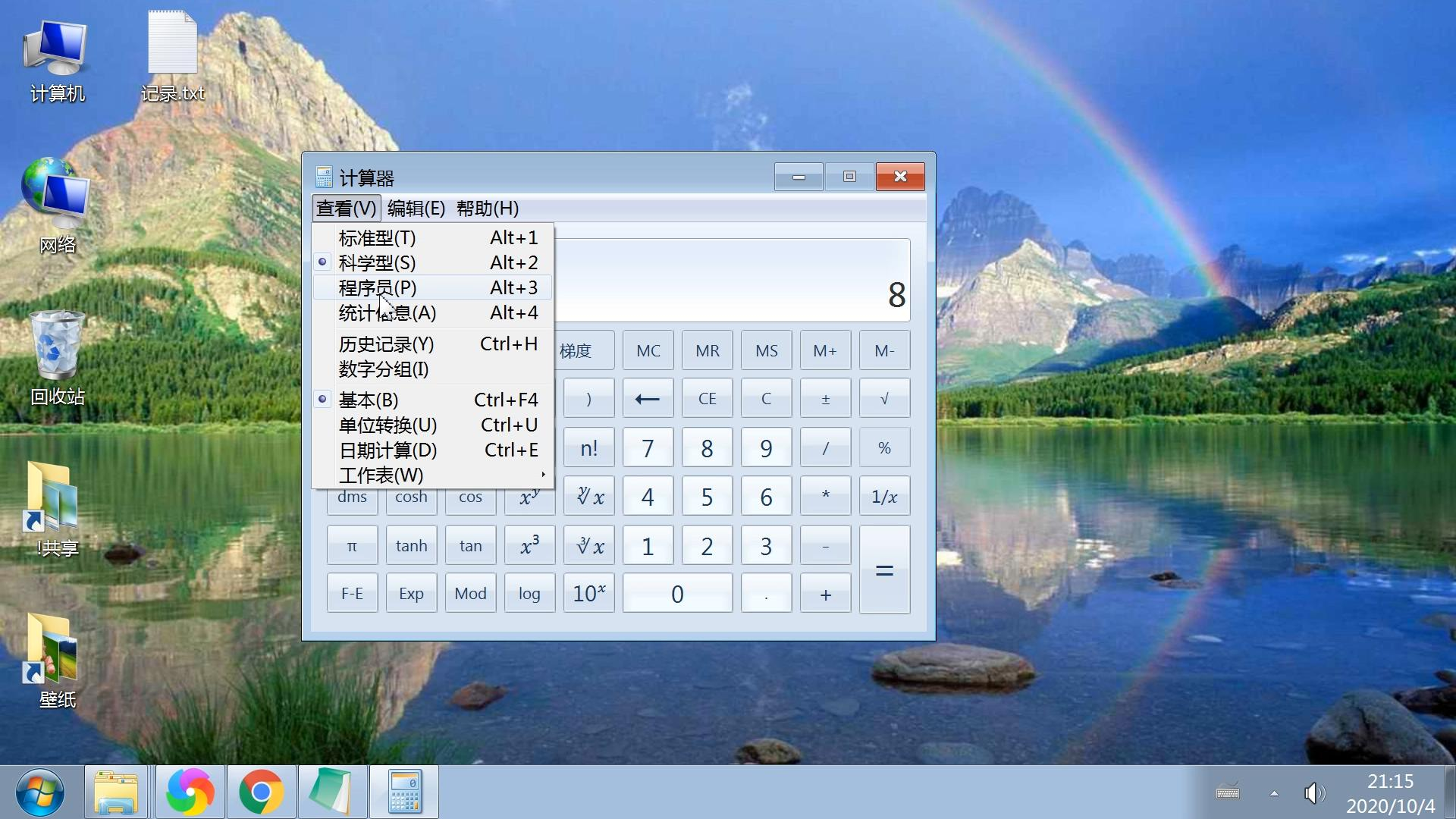菜单中的程序员选项我们点一下。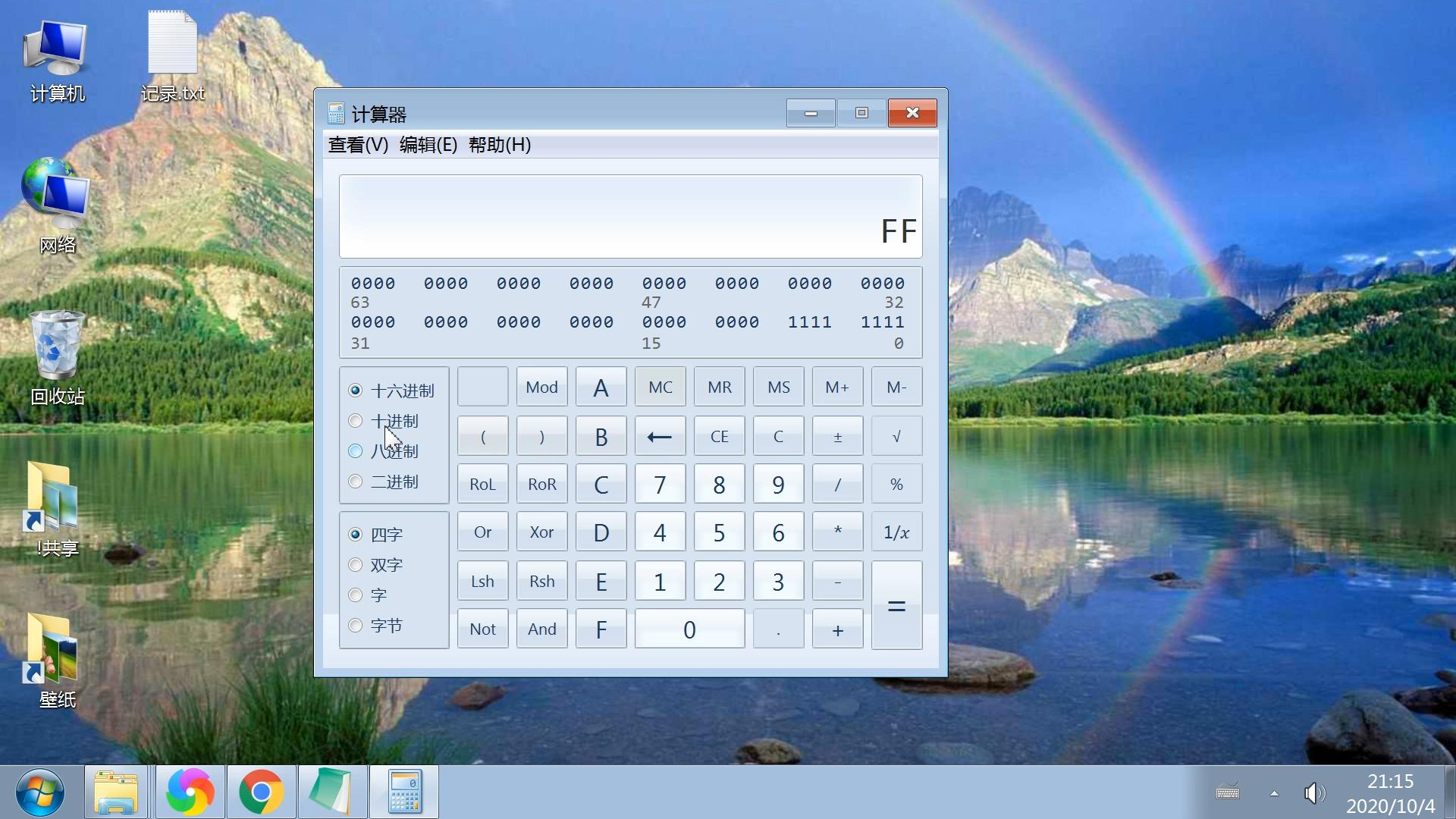在程序员这个计算器中，可以对一些数据进行逻辑运算，比如与，或，异或等一些运算，还可以进行十六进制八进制二进制等一些转换。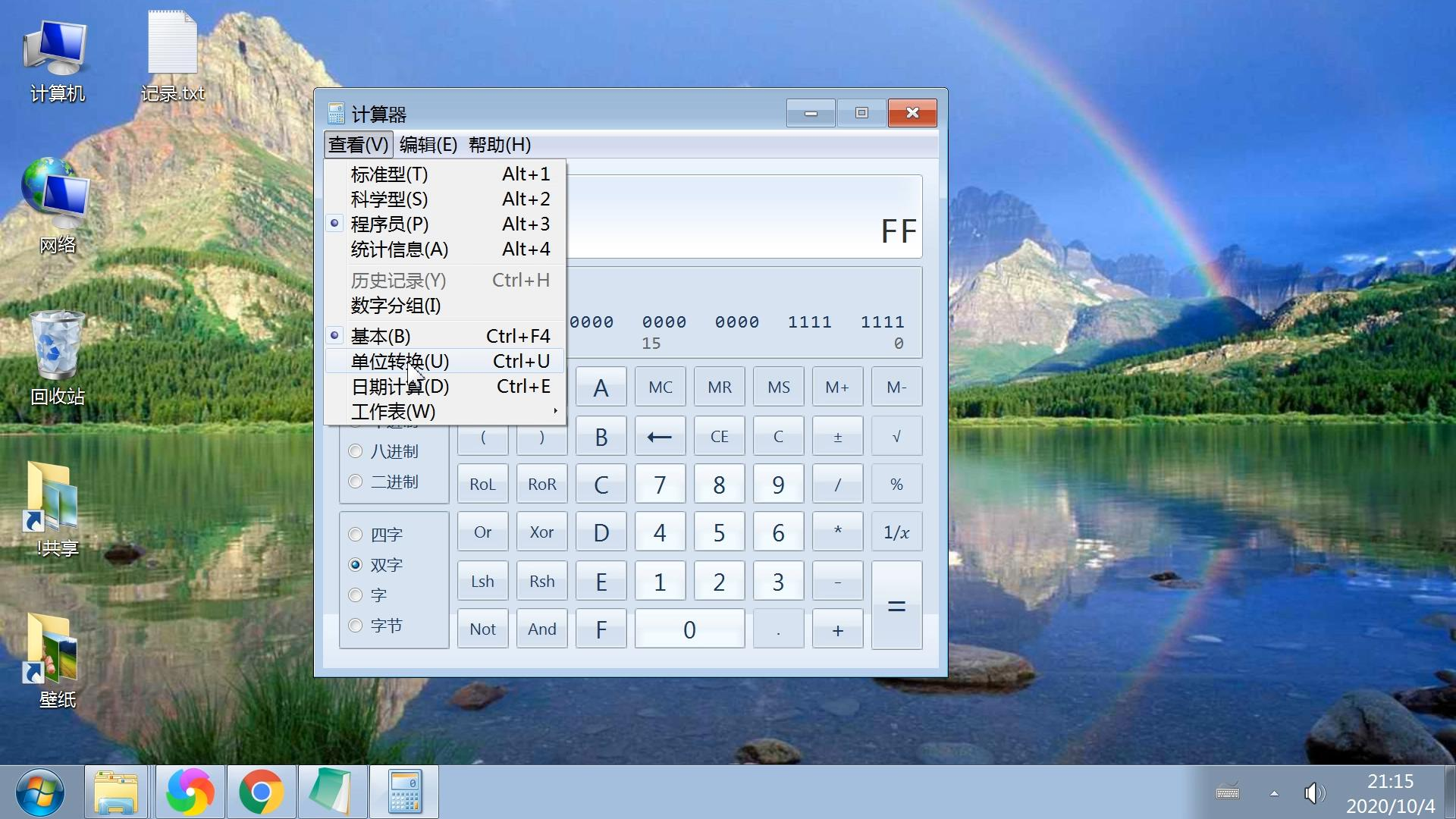我们再看一下菜单中的单位转换，点一下。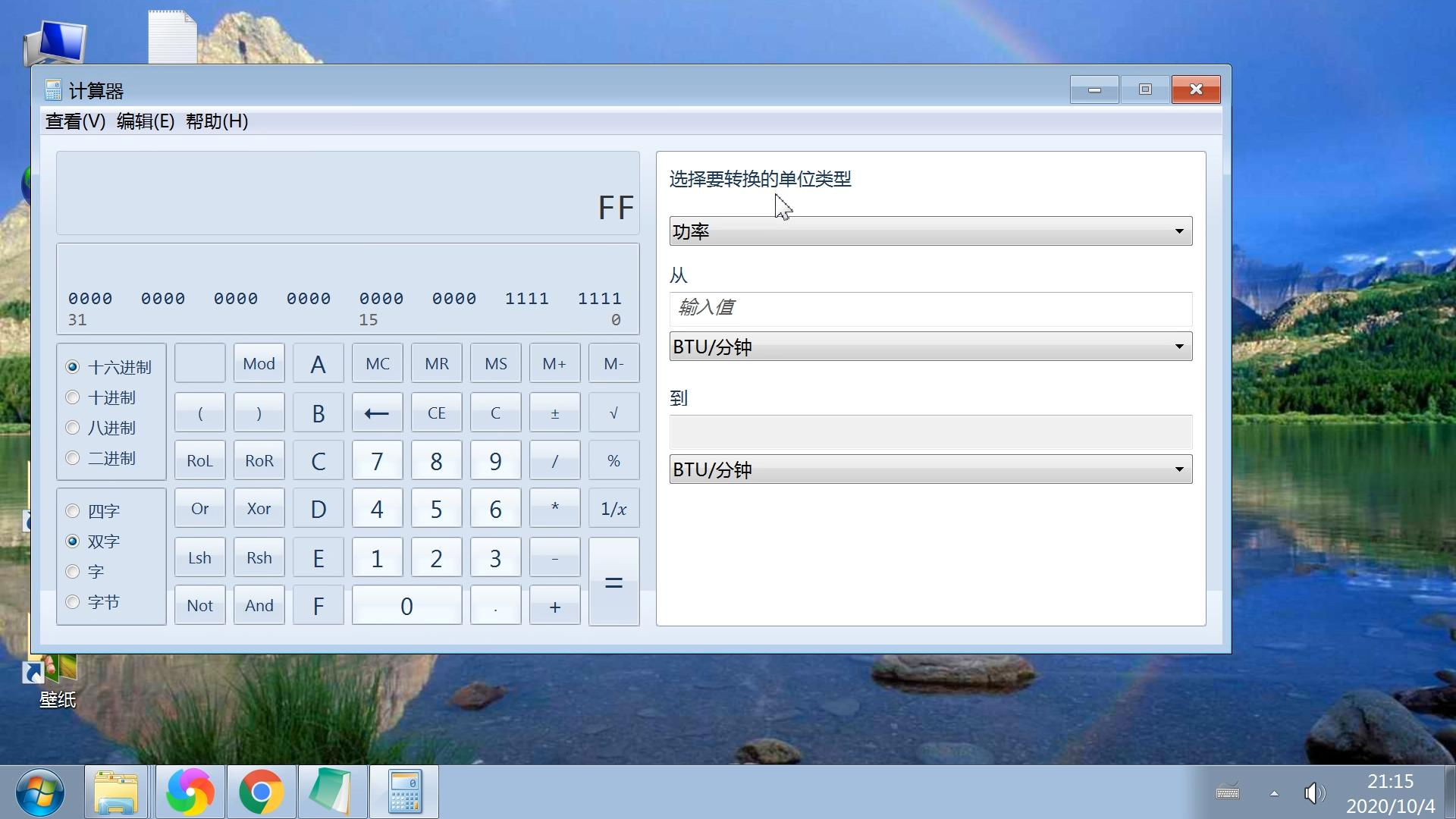单位转换包含了很多单位转换，右侧有一个菜单，点一下可以根据需要计算的单位进行转换。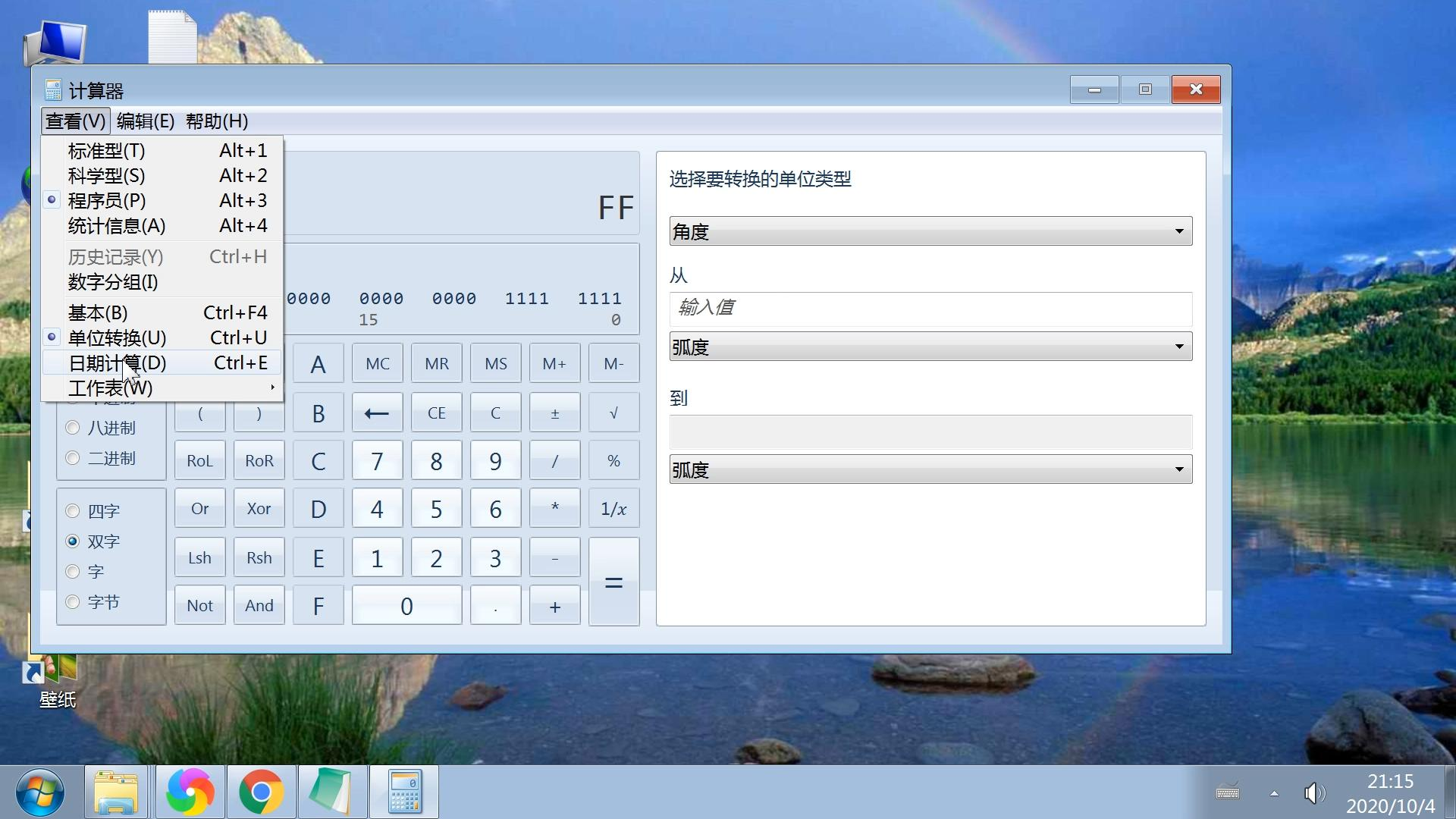菜单中左下方还有一个日期计算，点一下。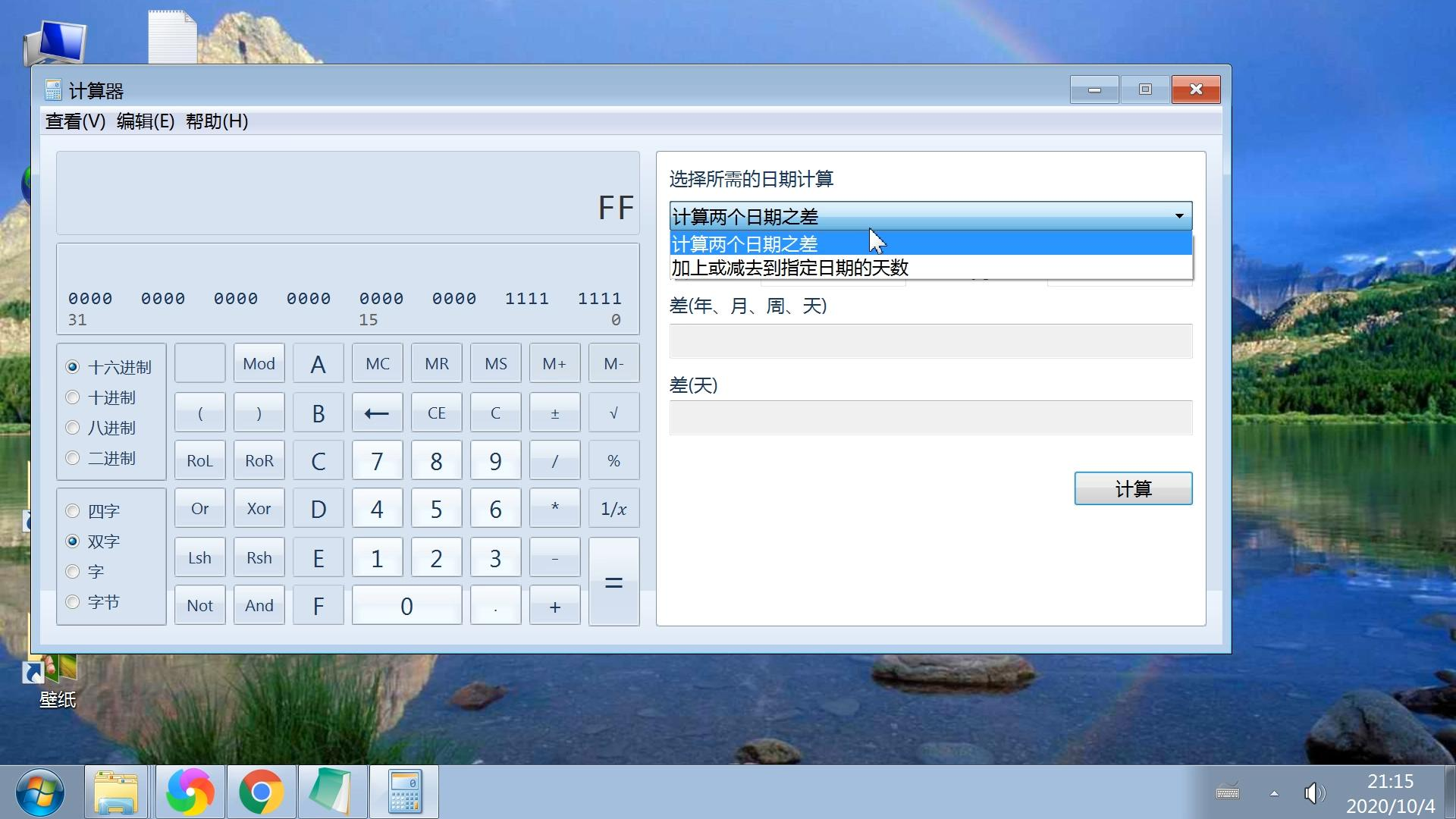这个就是日期计算，计算器中还有一些其他的选项，大家可以也可以测试查看一下。
感谢观看本篇文章，希望对你有所帮助，本文由老盖聊技术原创，本文是出于爱心帮助人的目的所写，请随意分享转载，感谢正能量传播。返回搜狐，查看更多
责任编辑：

展开全文• ## 异或运算

万次阅读 多人点赞 2017-11-17 18:45:11
异或运算https://www.lijinma.com/blog/2014/05/29/amazing-xor/
什么是异或？
Wikipedia的解释：

在逻辑学中，逻辑算符异或（exclusive or）是对两个运算元的一种逻辑析取类型，符号为 XOR 或 EOR 或 ⊕（编程语言中常用^）。但与一般的逻辑或不同，异或算符的值为真仅当两个运算元中恰有一个的值为真，而另外一个的值为非真。转化为命题，就是：“两者的值不同。”或“有且仅有一个为真。”

定义：

1 ⊕ 1 = 0
0 ⊕ 0 = 0
1 ⊕ 0 = 1
0 ⊕ 1 = 1

真值表：
Y B = 0 B = 1   A = 0 0 1   A = 1 1 0
表达式：
Y = A’ · B + A · B’

解释：我使用·作为与，我使用+作为或，我使用'作为否(本来应该使用头上一横，但是太难编辑了，就使用了'）；

异或有什么特性？
根据定义我们很容易获得异或两个特性：

恒等律：X ⊕ 0 = X 归零律：X ⊕ X = 0

然后我们使用真值表可以证明：
（1）交换律

1
2
3
A ⊕ B = A' · B + A · B'

B ⊕ A = B' · A + B · A'

因为·与和+或两个操作满足交换律，所以：
A ⊕ B = B ⊕ A
（2）结合律

1
2
3
4
5
6
7
8
9
10
11
12
13
(A ⊕ B) ⊕ C

= (A' · B + A · B') ⊕ C

= (A' · B + A · B')' · C + (A' · B + A · B') · C '

= ((A' · B)' · (A · B')')· C + A' · B · C ' + A · B' · C '

= ((A + B') · (A' + B))· C + A' · B · C ' + A · B' · C '

= (A · B + A' · B') · C + A' · B · C ' + A · B' · C '

= A · B · C + A' · B' · C + A' · B · C ' + A · B' · C '


你可以使用同样推导方法得出（请允许我偷懒一下，数学公式敲起来不容易 +_+）：

1
2
3
A ⊕ (B ⊕ C)

= A · B · C + A' · B' · C + A' · B · C ' + A · B' · C '

证明过程中使用了如下几个方法（·与 +或 '否）：
·与 +或交换律：

1
2
3
A · B = B · A

A + B = B + A

·与 +或结合律：

1
2
3
(A · B) · C = A · (B · C)

(A + B) + C = A + (B + C)　

·与 +或分配律：

1
2
3
A · (B + C)= A · B + A · C

A + B · C = (A + B) · (A + C)

摩尔定理：

1
2
3
(A · B)' = A' + B'

(A + B)' = A' · B'

结论：

交换律：A ⊕ B = B ⊕ A 结合律：A ⊕ (B ⊕ C) = (A ⊕ B) ⊕ C

有了归零率和结合律，我们就可以轻松证明：

自反：A ⊕ B ⊕ B = A ⊕ 0 = A

可能这些特性会很顺其自然的理解，但是如果你在解决问题的时候，你可能会忘记异或的这些特性，所以适当的应用可以让我们加深对异或的理解；

1
2
3
4
A ⊕ 1 = A';
A ⊕ 0 = A;
A ⊕ A = 0;
A ⊕ A' = 1;

异或有什么神奇之处（应用）？

说明：以下的的异或全部使用符号^

可能你已经被乱七八糟的公式和演算搞的有点烦了，不就是很简单的异或运算吗？还解释的那么复杂，嘿嘿，不要着急，打好了基础，你就站在了巨人的肩膀，让我们开始异或的神奇之旅吧；
（1）快速比较两个值
先让我们来一个简单的问题；判断两个int数字a，b是否相等，你肯定会想到判断a - b == 0，但是如果判断a ^ b == 0效率将会更高，但是为什么效率高呢？就把这个给你当家庭作业吧，考虑下减法是如何实现的； 让我们看看ipv6中的比较；

1
2
3
4
5
6
7
static inline int ipv6_addr_equal(const struct in6_addr *a1, const struct in6_addr *a2)
{
}


（2）在汇编语言中经常用于将变量置零：xor a，a；
（3）我们可以使用异或来使某些特定的位翻转，因为不管是0或者是1与1做异或将得到原值的相反值；
0 ^ 1 = 1
1 ^ 1 = 0
例如：翻转10100001的第6位， 答案：可以将该数与00100000进行按位异或运算;10100001 ^ 00100000 = 10000001
我们给出一段常用的代码：

1
2
3
unsigned int a, b, mask = 1 << 6;
a = 0xB1; // 10100001
b = a ^ mask; /* flip the 6th bit */


（4）我们使用异或来判断一个二进制数中1的数量是奇数还是偶数
例如：求10100001中1的数量是奇数还是偶数； 答案：1 ^ 0 ^ 1 ^ 0 ^ 0 ^ 0 ^ 0 ^ 1 = 1,结果为1就是奇数个1，结果为0就是偶数个1； 应用：这条性质可用于奇偶校验（Parity Check），比如在串口通信过程中，每个字节的数据都计算一个校验位，数据和校验位一起发送出去，这样接收方可以根据校验位粗略地判断接收到的数据是否有误
（5）校验和恢复
校验和恢复主要利用的了异或的特性：IF a ^ b = c THEN a ^ c = b 应用：一个很好的应用实例是RAID5，使用3块磁盘（A、B、C）组成RAID5阵列，当用户写数据时，将数据分成两部分，分别写到磁盘A和磁盘B，A ^ B的结果写到磁盘C；当读取A的数据时，通过B ^ C可以对A的数据做校验，当A盘出错时，通过B ^ C也可以恢复A盘的数据。
RAID5的实现比上述的描述复杂多了，但是原理就是使用 异或，有兴趣的同学看下RAID5
（6）经典题目：不使用其他空间，交换两个值

1
2
3
a = a ^ b;
b = a ^ b; //a ^ b ^ b = a ^ 0 = a;
a = a ^ b;


这个题目就不用解释了吧，太大众题目了，哈哈，但是非常好的使用的了异或的特性；
（7）面试题：互换二进制数的奇偶位；
题目：写一个宏定义，实现的功能是将一个int型的数的奇偶位互换，例如6的2进制为00000110，(从右向左)第一位与第二位互换，第三位与第四位互换，其余都是0不需要交换，得到00001001，输出应该为9；
思路：我们可以把我们的问题分为三步（难道这也是分治法吗 -。-），第一步，根据原值的偶数位获取到目标值的奇数位，并把不需要的位清零；第二步，根据原值的奇数位获取到目标值的偶数位，并把不需要的位清零；第三步：把上述两个残缺的目标值合并成一个完整的目标值；
代码为：

1
2
3
4
5
6
7
8
9
//假设 int 占两个字节，16位；
#include<iostream>
#include<string>
using namespace std;
#define N(n) ((n<<1)&(0xAAAA))|((n>>1)&(0x5555))
void main(){
int k = N(6);
cout << k << endl;
}


解释： 1.为简化说明，我们以4位二进制码为例，0xAAAA 我们用 1010 代替；0x5555 我们用 0101 代替； 2.(n<<1)&(1010) 把n先左移1位，再与1010做与运算，只保留移位之后的偶数位的值，奇数位全为0，实际上是只保留了n的奇数位的值，并把它们交换到了偶数位上。比如 n = 0110 , n<<1 = 1100, (n<<1) & 1010 = 1000 ; 3.(n>>1)&(0101) 把n右移一位，再与 0101 做与运算，只保留移位之后的奇数位的值，偶数位全为0，实际是只保留n 的偶数位的值，并把它们交换到对应的奇数位上。n = 0110； n>>1 = 0011； (n>>1) & 0101 = 0001； 4.最后做或运算（相加），得到1001。
（7）最最常出现的面试题：一个整型数组里除了N个数字之外，其他的数字都出现了两次，找出这N个数字；
比如，从{1, 2, 3, 4, 5, 3, 2, 4, 5}中找出单个的数字： 1
让我们从最简单的，找一个数字开始；
题目：（LeetCode 中通过率最高的一道题） Single Number: Given an array of integers, every element appears twice except for one. Find that single one. Note:Your algorithm should have a linear runtime complexity. Could you implement it without using extra memory? 思路： 拿到这个题目，本能的你会使用排序（数字文字我们常常需要排序），排序后可以来判断是否数字成对出现，思路很明显，但是排序的算法上限是 O(nlogn)，不符合题目要求；
学习了强大的异或，我们可以轻松的使用它的特性来完成这道题目： （1）A ^ A = 0; （2）异或满足交换律、结合律； 所有假设有数组：A B C B C D A 使用异或：

1
2
3
4
5
A ^ B ^ C ^ B ^ C ^ D ^ A
= A ^ A ^ B ^ B ^ C ^ C ^ D
= 0 ^ 0 ^ 0 ^ D
= 0 ^ D
= D


是不是很神奇？时间复杂度为O(n)，当然是线性的，空间复杂度O(1)；
代码：

1
2
3
4
5
6
7
8
9
10
11
12
13
14
class Solution {
public:
int singleNumber(int A[], int n) {
//特殊情况1,2
if(n<=0) return -1;
if(n==1) return A;

int result = 0;
for (int i = 0; i < n; i ++) {
result = result ^ A[i];
}
return result;
}
};


接下来让我们增加一些难度：
题目：一个整型数组里除了两个数字之外，其他的数字都出现了两次。请写程序找出这两个只出现一次的数字？
思路： 第一步：肯定还是像我们上面的解法一样，所有数进行异或，不过最终得到的结果是 a 和 b（假设 a 和 b 是落单的数字）两个值的异或结果 aXORb，没有直接得到 a 和 b 的值；
第二步：想办法得到 a 或者 b，假设 aXORb 为 00001001（F肯定不为0），根君 aXORb 的值我们发现，值为1的位（比如从右向左第一位）表示在此位上 a 和 b 的值不同；所以，根据这个特点，我们找出来所有第一位为1的数进行异或，得到的就是 a 或者 b；
第三步：aXORb = a ^ b，假设我们已经找到了 a，根据异或特性，我们知道，b = aXORb ^ a；这样我们就可以找出 b；所以我们只需要循环两次；
这样我们的时间复杂度是 O(n)，空间复杂度是 O(1) 代码：

1
2
3
4
5
6
7
8
9
10
11
12
13
14
15
16
17
18
19
20
21
22
23
24
25
26
27
28
29
30
31
32
33
34
35
36
#include <iostream>
#include <assert.h>
using namespace std;

int getFirstOneBit(int num) //输出 num 的低位中的第一个 1 的位置
{
return num & ~(num - 1);  // num 与 -num 相与找到
}

void findTwo(int *array, int length){
int aXORb = 0;
int firstOneBit = 0;
int a = 0;
int b = 0;
for (int i = 0; i < length; i++) {
aXORb ^= array[i];
}
assert(aXORb != 0); //保证题目要求，有两个single的数字
firstOneBit = getFirstOneBit(aXORb);
for (int i = 0; i < length; ++i) {
if(array[i] & firstOneBit) {
a ^= array[i];
}
}
b = aXORb ^ a;
cout << "a: " << a << endl;
cout << "b: " << b << endl;
}

int main()
{
int array1[] = {2, 5, 8, 2, 5, 8, 6, 7};
findTwo(array1, 8);
return 0;
}


接下来让我们再增加一些难度：
题目：一个整型数组里除了三个数字之外，其他的数字都出现了两次。请写程序找出这两个只出现一次的数字？
思路：
第一步：肯定还是像我们上面的解法一样，所有数进行异或，不过最终得到的结果是 a、b 和 c（假设 a、b 和 c 是落单的数字）三个值的异或结果 aXORbXORc，没有直接得到 a、b 和 c 的值；
第二步：想办法得到 a、b 和 c 中的一个，让偶们把问题简化一下；
假设一个数组中有3个不同的数字 a、b 和 c，已知 aXORbXORc = a ^ b ^ c ，求 a、b 和 c 。
思路： 1. 根据题目 aXORbXORc ^ a = b ^ c; aXORbXORc ^ b = a ^ c; aXORbXORc ^ c = a ^ b; 因为：(b ^ c) ^ (a ^ c) ^ (a ^ b) = 0; 所以：(aXORbXORc ^ a) ^ (aXORbXORc ^ b) ^ (aXORbXORc ^ c) = 0;
下一步是关键： 假设 X ^ Y ^ Z = 0，则 X Y Z 三个数的低位第一位为1的位置两个相同，一个不同； 比如 X: 00001000, Y: 00000100, Z: 00001100 Y和Z的低位第一位都是00000100， X的低位第一位是00001000； 这一步可以使用倒推法证明： 已知：三个数的低位第一位为1的位置有三种情况，一种就是全相同，一种就是两个不同，一个不同，一种就是三个不同； （1）如果是全相同，则 X ^ Y ^ Z != 0 (1 ^ 1 ^ 1 = 1)，与前提X ^ Y ^ Z = 0矛盾，不成立； （2）如果三个不同，则 X ^ Y ^ Z != 0 (1 ^ 0 ^ 0 = 1)，与前提X ^ Y ^ Z = 0矛盾，不成立； 所以结果是：两个不同，一个不同   (aXORbXORc ^ a) ^ (aXORbXORc ^ b) ^ (aXORbXORc ^ c) = 0; 所以三个数(aXORbXORc ^ a)、(aXORbXORc ^ b) 和 (aXORbXORc ^ c) 的低位第一位为1的位置两个相同，一个不同；那么我们获取到这三个数的低位第一位为1的位置后，进行异或并取低位第一位为1的位置，就可以找到三个中“一个不同”的低位第一位为1的位置，假设这个值为 firstOneBit。   遍历这三个数(aXORbXORc ^ a)、(aXORbXORc ^ b) 和 (aXORbXORc ^ c)，如果发现某个数异或 aXORbXORc 等于 firstOneBit，这个数就是“一个不同”的那个数；   找到了一个数，剩下的两个数，我们就可以通过上面的方法找出来；
第三步：完成了第二步的简化题，我们回到我们的问题，我们的问题比简化的问题多了一个成对的干扰数据，我们可以使用异或要去除干扰数据（记住，我们这个题目都是用异或i去除干扰数据的）；
这样我们的时间复杂度还是 O(n)，空间复杂度是 O(1)
代码如下：

1
2
3
4
5
6
7
8
9
10
11
12
13
14
15
16
17
18
19
20
21
22
23
24
25
26
27
28
29
30
31
32
33
34
35
36
37
38
39
40
41
42
43
44
45
46
47
48
49
50
51
52
53
54
55
56
57
58
59
60
61
#include <iostream>
#include <assert.h>
using namespace std;

int getFirstOneBit(int num) //输出 num 的低位中的第一个 1 的位置
{
return num & ~(num - 1);  // num 与 -num 相与找到
}

void findTwo(int *array, int length){
int aXORb = 0;
int firstOneBit = 0;
int a = 0;
int b = 0;
for (int i = 0; i < length; i++) {
aXORb ^= array[i];
}
assert(aXORb != 0); //保证题目要求，有两个single的数字
firstOneBit = getFirstOneBit(aXORb);
for (int i = 0; i < length; ++i) {
if(array[i] & firstOneBit) {
a ^= array[i];
}
}
b = aXORb ^ a;
cout << "a: " << a << endl;
cout << "b: " << b << endl;
}

int findOne(int *array, int length) {
int aXORbXORc = 0;
int c = 0;
int firstOneBit = 0;
for (int i = 0; i < length; ++i) {
aXORbXORc ^= array[i];
}

for (int i = 0; i < length; ++i) {
firstOneBit ^= getFirstOneBit(aXORbXORc ^ array[i]); //使用异或会排除掉不相干的元素
}
// firstOneBit = getFirstOneBit(a ^ b) ^ getFirstOneBit(a ^ c) ^ getFirstOneBit(b ^ c);

firstOneBit = getFirstOneBit(firstOneBit); //获取到最低位下面要用

for (int i = 0; i < length; ++i) {
if (getFirstOneBit(aXORbXORc ^ array[i]) == firstOneBit) {
c ^= array[i]; //使用异或会排除掉不相干的元素
}
}
cout << "c: " << c << endl;
return c;
}

int main()
{
int array1[] = {2, 5, 8, 2, 5, 8, 6, 7, 1};
int c = findOne(array1, 9);
int array2[] = {2, 5, 8, 2, 5, 8, 6, 7, 1, c}; //为了更好重用函数，我重新定义了一个数组让大家理解
findTwo(array2, 10);
return 0;
}


写这篇文档参考了《离散数学与应用》课本，参考了别人多个博客，如果我参考了你的博客，但没有注明出处，请联系告知，有错误的地方，希望可以指出来，也希望大家有更多的补充，非常感谢。
参考：
http://zh.wikipedia.org/wiki/%E9%80%BB%E8%BE%91%E5%BC%82%E6%88%96
http://yjq24.blogbus.com/logs/41863963.html
http://wzw19191.blog.163.com/blog/static/131135470200992610551971/
http://kapok.blog.51cto.com/517862/129941
http://blog.csdn.net/huxian370/article/details/8024416
http://www.cnblogs.com/Ivony/archive/2009/07/23/1529254.html
http://blog.chinaunix.net/uid-20937170-id-3407361.html
http://blog.csdn.net/yfkiss/article/details/11775569
http://blog.sina.com.cn/s/blog_88c9ddc50101810p.html
http://blog.csdn.net/pathuang68/article/details/7567027
http://blog.csdn.net/qingen1/article/details/12656763
展开全文• ## 二进制逻辑运算

千次阅读 2017-09-29 16:14:44
逻辑加法（“或”运算）逻辑加法通常用符号“+”或“∨”来表示。逻辑加法运算规则如下： 0+0=0， 0∨0=0 0+1=1， 0∨1=1 1+0=1， 1∨0=1 1+1=1， 1∨1=1 从上式可见，逻辑加法有“或”的意义。也就是说，在...
逻辑加法（“或”运算）
逻辑加法通常用符号“+”或“∨”来表示。逻辑加法运算规则如下：  0+0=0， 0∨0=0  0+1=1， 0∨1=1  1+0=1， 1∨0=1  1+1=1， 1∨1=1  从上式可见，逻辑加法有“或”的意义。也就是说，在给定的逻辑变量中，A或B只要有一个为1，其逻辑加的结果就为1；只有当两者都为0时逻辑加的结果才为0。
逻辑乘法（“与”运算）
逻辑乘法通常用符号“×”或“∧”或“·”来表示。逻辑乘法运算规则如下：  0×0=0， 0∧0=0， 0·0=0  0×1=0， 0∧1=0， 0·1=0  1×0=0， 1∧0=0， 1·0=0  1×1=1， 1∧1=1， 1·1=1  不难看出，逻辑乘法有“与”的意义。它表示只当参与运算的逻辑变量都同时取值为1时，其逻辑乘积才等于1。
逻辑否定（”非”运算）
逻辑非运算又称逻辑否运算。其运算规则为：  0=1 “非”0等于1  1=0 “非”1等于0
异或逻辑运算（“半加”运算）
异或运算通常用符号”⊕”表示，其运算规则为：  0⊕0=0 0同0异或，结果为0  0⊕1=1 0同1异或，结果为1  1⊕0=1 1同0异或，结果为1  1⊕1=0 1同1异或，结果为0  即两个逻辑变量相异，输出才为1

异或口诀：异1同0

百科
展开全文• 与、或、异或运算 1.与运算（&） 参加运算的两个数据，按二进制位进行“与”运算。 运算规则：0&0=0;0&1=0;1&0=0;1&1=1; 即：两位同时为“1”，结果才为“1”，否则为0 例如：3&5即 ...
与、或、异或运算
1.与运算（&）
参加运算的两个数据，按二进制位进行“与”运算。
运算规则：0&0=0;   0&1=0;    1&0=0;     1&1=1;
即：两位同时为“1”，结果才为“1”，否则为0
例如：3&5  即 0000 0011 & 0000 0101 = 0000 0001   因此，3&5的值得1。
例如：9&5  即 0000 1001 (9的二进制补码)&00000101 (5的二进制补码) =00000001 (1的二进制补码)可见9&5=1。
2.或运算（|）

参加运算的两个对象，按二进制位进行“或”运算。

运算规则：0|0=0；   0|1=1；   1|0=1；    1|1=1；

即 ：参加运算的两个对象只要有一个为1，其值为1。

例如:3|5　即 0000 0011 | 0000 0101 = 0000 0111   因此，3|5的值得7。
例如：9|5可写算式如下： 00001001|00000101 =00001101 (十进制为13)可见9|5=13
3.异或运算（^）

参加运算的两个数据，按二进制位进行“异或”运算。

运算规则：0^0=0；   0^1=1；   1^0=1；   1^1=0；
即：参加运算的两个对象，如果两个相应位为“异”（值不同），则该位结果为1，否则为0。

例如：9^5可写成算式如下： 00001001^00000101=00001100 (十进制为12)可见9^5=12

常用运算：（二进制赋值、取值、左移、右移、求余数、向上取整、向下取整）
1.赋值1 -> 或运算（|）
   例如：40 | (1<<1)  = 42

0010 1000

| 0000 0010

------------------

0010 1010
2.赋值0 -> 与非运算（&~）
例如： 42 & ~(1<<1) = 40

0010 1010 & ~0000 0010

==》

0010 1010

& 1111 1110
---------------

0010 1000
3.取值 -> 与运算（&）
例如：42 & 1<<1 = 2

0010 1010

& 0000 0010

-----------------

0000 0010
4.乘以2 -> 左移一位（ << 1）
例如：43 * 2 = 43 << 1 = 86

0010 1011 >> 0101 0110 = 86
5.除以2 -> 右移一位（ >> 1）
例如：43 / 2 = 43 >> 1

0010 1011 >> 0001 0101 = 21
6.除以2求余数 -> 与1（ &1）
例如：43 % 2 = 43 & 1 = 1

0010 1011

& 0000 0001

---------------

0000 0001
7.向上取整
int a , int b => (a + b - 1) / b

比如：

a = 8, b = 3 => 2;

a = 9, b = 3 => 3;

a = 10, b = 3 => 4;
8.向下取整
int a , int b => a / b



展开全文C
• 二、二进制的加减运算 十进制是逢十进一，二进制是逢二进一，十进制的世界单个数字最大为9，二进制的世界单个数字最大为1。 10010011 10101001 ---------------- 100111100 思考一个问题，计算机只能保存0和1...java
• 适合软硬件开发人员的计算器二进制运算强大，支持64位。 采用表达式驱动，输入表达式便即时显示结果，抛弃传统计算器繁琐的按钮，表达式可复制粘贴、回调使用，可以任意复杂组合。 支持二进制串直接运算，如0b1101...
• 学习背景：最近在看很多JAVA类的源码，遇到了很多的位运算，所以系统的学习了下有关二进制的知识。 首先，看一下JAVA中的基本数据的字节(Byte)长度和bit长度： 基本数据类型 字节Byte bit byte 1字节 8位 ...java 补码
• 异或运算异或，英文为exclusive OR，缩写成xor。异或(eor)是一个数学运算符。它应用于逻辑运算。异或的数学符号为“⊕”，计算机符号为“eor”。1.归零律：a⊕a=02.恒等律：a⊕0=a3.交换律：a⊕b=b⊕a4.结合律：a⊕b...
• 异或是2进制运算，计算机内部是二进制运算，我们看到十进制是转换后的结果 1.相同为0，不同为1 2.0与任何数异或等于任何数 3.a ^ b ^ b = b ^ a ^ b = b ^ b ^ a = a(两次异或会相消)很重要可以作为基础对称加密...算法
• 专为程序员打造的计算器二进制运算强大，支持64位。 采用表达式驱动，输入表达式便即时显示结果，抛弃传统计算器繁琐的按钮，表达式可粘贴或回调重复使用。 支持二进制串直接运算，如0b1101 & 0b0011= 0b0001。 ...
• 满意答案dawlqa2013.11.10采纳率：45%等级：12已帮助：7560人将你输入的数转换成二进制进行以下的运算,再转换成十进制输出1. and运算(与)and运算通常用于二进制取位操作，例如一个数 and 1的结果就是取二进制的最...
• ## 论逻辑异或运算xor

千次阅读 2013-08-07 17:21:38
xor称作异或运算 怎么个异或法呢 很多人都没有搞清 至少，我刚开如是这样的 知道是或运算就行了 知道xor可以生成一个值，并且通过这值用同样的方式还原回去 最后 要得到结果，大都是用计算器算一下 作为...
• 1.在c语言中异或的用法是什么异或是一个数学运算符。它应用于逻辑运算。在【布尔值运算】中：真异或假的...异或也叫半加运算，其运算法则相当于不带进位的二进制加法：二进制下用1表示真，0表示假，则异或运算法则...
• nowcoder 213756，二进制二进制运算
• 1. 按位与运算 按位与运算符"&... 例如：9&5可写算式如下： 00001001 (9的二进制补码)&00000101 (5的二进制补码) 00000001 (1的二进制补码)可见9&5=1。 按位与运算通常用来对某些位清0或保留某些位。例如把a 的高八位清存储 input c 测试
• 一款对16进制串进行异或校验的工具，对硬件开发有帮助。
• 虽然很早就接触了二进制，却一直没有正视这个问题，阅读《计算机科学导论》的时候，基本上是跳过了这一部分，总是以“这么基础的东西，反正工作中基本用不上”的理由给搪塞过去。最近正在阅读《编码》和《程序员的...
• 提纲 ...电脑的二进制其首位是符号位（用来表示二进制数的正负），首位“1”表示负数，“0”表示正数 电脑用二进制的优点 二进制状态简单 可靠性，稳定性高 运算规则简单，设计简单 通用...源码、反码、补码
• 二进制转十进制计算器二进制：十进制：进制：进制：转换说明：上面第一行可将二进制转换为十进制，第二行可以将任意进制转换为任意进制。64个基数为："[emailprotected]"注意：本工具只是各种进制计数法的数之间的...
• 运算是直接在二进制层面0和1上操作的，所以它的操作效率非常高，比如正数除法可可以通过右移实现，而且它的效率比用除法高很多，java中很多类源码需要运算时常能看到作者用的是位运算来操作。 2. 异或、左移、右移...原码、补码、反码 数组中出现一次的数字数学计算
• 数据宽度_逻辑运算 文章目录数据宽度_逻辑运算一、数据宽度二、逻辑运算32位通用寄存器补充 ...二进制是为了方便我们学习和使用而引入的。是人为规定的。 汇编语言常用的数据宽度： 位(Bit)： 表示一个
• 共回答了25个问题采纳率：88%将你输入的数转换成二进制进行以下的运算,再转换成十进制输出1.and运算(与)and运算通常用于二进制取位操作,例如一个数 and 1的结果就是取二进制的最末位.这可以用来判断一个整数的奇偶,...
• 概述二进制位运算符用于直接对二进制位进行计算，一共有7个。二进制或运算符(or)：符号为|，表示若两个二进制位都...异或运算符(xor)：符号为^，表示若两个二进制位不相同，则结果为1，否则为0。左移运算符(left shi...
• 现代计算机的各个部件到底是如何通过逻辑电路构成的呢 半加器 我们说过了门电路 看似简单的三种门电路却是组成了整个逻辑电路的根基 真值表--其实就是根据输入输出状态枚举罗列出来的所有可能 ...第步:把表...
• （1）从上节可知，对寄存器特定位进行置1或者清0或者取反，关键性的难点在于事先构建一个特别的数，这个数和原来的值进行位与、位或、位异或等操作，即可达到我们对寄存器操作的要求。 （2）解法1：用工具软件或者...
• (1)从上节可知，对寄存器特定位进行置1或者清0或者取反，关键性的难点在于要事先构建一个特别的数，这个数和原来的值进行位与、位或、位异或等操作，即可达到我们对寄存器操作的要求。 (2)解法1：用工具软件或者...
• 一、常量的概述与使用 和C++一样，Java中的常量在定义之后它的值就不可以发生改变。 java中的常量分为字面值常量和自定义常量 字面值常量有以下几种 ...即逢二进一，在计算机中机器码就是二进制表示的，因为.补码 数据类型 Java运算符
• 1、 AND 逻辑与运算 AND为用于评估两个条件表达式（真/假）的函数返回值为True、False 或者 1、0 条件判断时，要同时满足才为真 举例 1 and 1 返回结果为1，也就是为真 1 and 0 返回结果为0, 也就是假 0 and 0 返回......